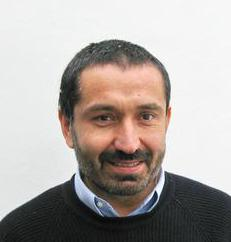# Mixed finite elements and adaptive schemes for eigenvalue problemsSpeaker:
Daniele Boffi
Affiliation:
Dipartimento di Matematica "F. Casorati", Università di Pavia
When:
Thursday 12th April 2018
Time:
14:00:00
Where:
Aula Consiglio VII Piano - Edificio 14, Dipartimento di Matematica POLITECNICO DI MILANO
Abstract:
We review a posteriori error analysis and adaptive schemes for the approximation of eigenvalue problems arising from partial differential equations. Our ultimate goal is the design of adaptive schemes for the approximation of the eigenmodes associated with Maxwell's equations. It is well known that the Maxwell eigenvalue problem can be analyzed with the help of suitable mixed formulations. Taking advantage of this remark, we can prove the convergence with optimal rate for the edge finite element approximation of the Maxwell eigenvalue problem. In three dimensions, the result is not a trivial extension of the analysis previously performed for the approximation of the Laplace eigenproblem in mixed form. Particular attention is paid to the case of multiple eigenvalues and clusters of eigenvalues. Contact: christian.vergara@polimi.it
Note:
Daniele Boffi is professor of Numerical Analysis at the University of Pavia. His research focuses on the finite element discretization of partial differential equation with particular emphasis on mixed finite elements. In particular, he is working on computational fluid dynamics, computational electromagnetism, and fluid-structure interactions. He is Director of the Doctoral School of the University of Pavia.